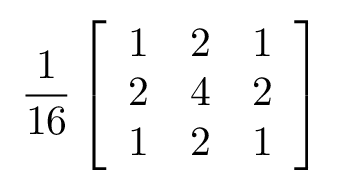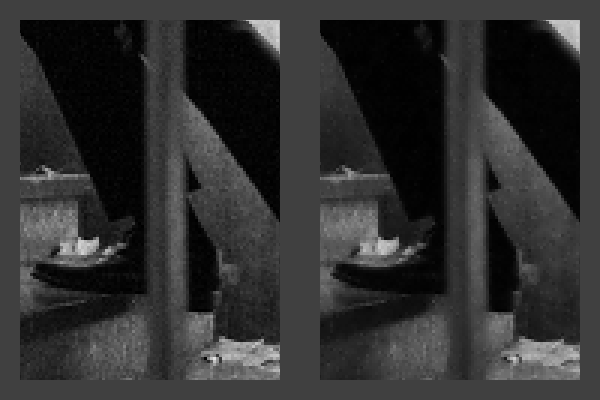A simple real-world example showing an image-processing Comonad
Published on November 27, 2014 under the tag haskell

## Introduction

Comonads are well-suited for image processing
– Pretty much everyone on the internet

Whenever Comonads come up, people usually mention the canonical example of evaluating cellular automata. Because many image processing algorithms can be modelled as a cellular automaton, this is also a frequently mentioned example.

However, when I was trying to explain Comonads to a friend recently, I couldn’t find any standalone example of how exactly this applies to image processing, so I decided to illustrate this myself.

I will not attempt to explain Comonads, for that I refer to Gabriel Gonzalez’ excellent blogpost. This blogpost is written in literate Haskell so you should be able to just load it up in GHCi and play around with it (you can find the raw `.lhs` file here).

``````{-# LANGUAGE BangPatterns #-}
import qualified Codec.Picture       as Juicy
import           Control.Applicative ((<\$>))
import           Data.List           (sort)
import           Data.Maybe          (fromMaybe, maybeToList)
import qualified Data.Vector         as V
import qualified Data.Vector.Generic as VG
import           Data.Word           (Word8)``````

## A simple image type

We need a simple type for images. Let’s use the great JuicyPixels library to read and write images. Unfortunately, we cannot use the image type defined in JuicyPixels, since JuicyPixels stores pixels in a Storable-based Vector.

We want to be able to store any kind of pixel value, not just `Storable` values, so we declare our own `BoxedImage`. We will simply store pixels in row-major order in a boxed `Vector`.

``````data BoxedImage a = BoxedImage
{ biWidth  :: !Int
, biHeight :: !Int
, biData   :: !(V.Vector a)
}``````

Because our `BoxedImage` allows any kind of pixel value, we get a straightforward `Functor` instance:

``````instance Functor BoxedImage where
fmap f (BoxedImage w h d) = BoxedImage w h (fmap f d)``````

Now, we want to be able to convert from a JuicyPixels image to our own `BoxedImage` and back again. In this blogpost, we will only deal with grayscale images (`BoxedImage Word8`), since this makes the image processing algorithms mentioned here a lot easier to understand.

``type Pixel = Word8  -- Grayscale``
``````boxImage :: Juicy.Image Juicy.Pixel8 -> BoxedImage Pixel
boxImage image = BoxedImage
{ biWidth  = Juicy.imageWidth image
, biHeight = Juicy.imageHeight image
, biData   = VG.convert (Juicy.imageData image)
}``````
``````unboxImage :: BoxedImage Pixel -> Juicy.Image Juicy.Pixel8
unboxImage boxedImage = Juicy.Image
{ Juicy.imageWidth  = biWidth boxedImage
, Juicy.imageHeight = biHeight boxedImage
, Juicy.imageData   = VG.convert (biData boxedImage)
}``````

With the help of `boxImage` and `unboxImage`, we can now call out to the JuicyPixels library:

``````readImage :: FilePath -> IO (BoxedImage Pixel)
case errOrImage of
Right (Juicy.ImageY8 img) -> return (boxImage img)
Right _                   ->
Left err                  ->
``````writePng :: FilePath -> BoxedImage Pixel -> IO ()
writePng filePath = Juicy.writePng filePath . unboxImage``````

## Focused images

While we can already write simple image processing algorithms (e.g. tone mapping) using just the `Functor` interface, the kind of algorithms we are interested in today need take a neighbourhood of input pixels in order to produce a single output pixel.

For this purpose, let us create an additional type that focuses on a specific location in the image. We typically want to use a smart constructor for this, so that we don’t allow focusing on an `(x, y)`-pair outside of the `piBoxedImage`.

``````data FocusedImage a = FocusedImage
{ piBoxedImage :: !(BoxedImage a)
, piX          :: !Int
, piY          :: !Int
}``````

Conversion to and from a `BoxedImage` is easy:

``````focus :: BoxedImage a -> FocusedImage a
focus bi
| biWidth bi > 0 && biHeight bi > 0 = FocusedImage bi 0 0
| otherwise                         =
error "Cannot focus on empty images"``````
``````unfocus :: FocusedImage a -> BoxedImage a
unfocus (FocusedImage bi _ _) = bi``````

And the functor instance is straightforward, too:

``````instance Functor FocusedImage where
fmap f (FocusedImage bi x y) = FocusedImage (fmap f bi) x y``````

Now, we can implement the fabled Comonad class:

``````class Functor w => Comonad w where
extract :: w a -> a
extend  :: (w a -> b) -> w a -> w b``````

The implementation of `extract` is straightforward. `extend` is a little trickier. If we look at it’s concrete type:

``extend :: (FocusedImage a -> b) -> FocusedImage a -> FocusedImage b``

We want to convert all pixels in the image, and the conversion function is supplied as `f :: FocusedImage a -> b`. In order to apply this to all pixels in the image, we must thus create a `FocusedImage` for every position in the image. Then, we can simply pass this to `f` which gives us the result at that position.

``````instance Comonad FocusedImage where
extract (FocusedImage bi x y) =
biData bi V.! (y * biWidth bi + x)

extend f (FocusedImage bi@(BoxedImage w h _) x y) = FocusedImage
(BoxedImage w h \$ V.generate (w * h) \$ \i ->
let (y', x') = i `divMod` w
in f (FocusedImage bi x' y'))
x y``````

Proving that this instance adheres to the Comonad laws is a bit tedious but not that hard if you make some assumptions such as:

``V.generate (V.length v) (\i -> v V.! i) = v``

We’re almost done with our mini-framework. One thing that remains is that we want to be able to look around in a pixel’s neighbourhood easily. In order to do this, we create this function which shifts the focus by a given pair of coordinates:

``````neighbour :: Int -> Int -> FocusedImage a -> Maybe (FocusedImage a)
neighbour dx dy (FocusedImage bi x y)
| outOfBounds = Nothing
| otherwise   = Just (FocusedImage bi x' y')
where
x'          = x + dx
y'          = y + dy
outOfBounds =
x' < 0 || x' >= biWidth bi ||
y' < 0 || y' >= biHeight bi``````

## Median filter

If you have ever taken a photo when it is fairly dark, you will notice that there is typically a lot of noise. We’ll start from this photo which I took a couple of weeks ago, and try to reduce the noise in the image using our Comonad-based mini-framework.

A really easy noise reduction algorithm is one that looks at a local neighbourhood of a pixel, and replaces that pixel by the median of all the pixels in the neighbourhood. This can be easily implemented using `neighbour` and `extract`:

``````reduceNoise1 :: FocusedImage Pixel -> Pixel
reduceNoise1 pixel = median
[ extract p
| x <- [-2, -1 .. 2], y <- [-2, -1 .. 2]
, p <- maybeToList (neighbour x y pixel)
]``````

Note how our Comonadic function takes the form of `w a -> b`. With a little intuition, we can see how this is the dual of a monadic function, which would be of type `a -> m b`.

We used an auxiliary function which simply gives us the median of a list:

``````median :: Integral a => [a] -> a
median xs
| odd len   = sort xs !! (len `div` 2)
| otherwise = case drop (len `div` 2 - 1) (sort xs) of
(x : y : _) -> x `div` 2 + y `div` 2
_           -> error "median: empty list"
where
!len = length xs``````

So `reduceNoise1` is a function which takes a pixel in the context of its neighbours, and returns a new pixel. We can use `extend` to apply this comonadic action to an entire image:

``extend reduceNoise1 :: FocusedImage Pixel -> FocusedImage Pixel``

Running this algorithm on our original picture already gives an interesting result, and the noise has definitely been reduced. However, you will notice that it has this watercolour-like look, which is not what we want.

## Blur filter

A more complicated noise-reduction filter uses a blur effect first. We can implement a blur by replacing each pixel by a weighted sum of its neighbouring pixels. At the edges, we just keep the pixels as-is.

This function implements the simple blurring kernel:``````blur :: FocusedImage Pixel -> Pixel
blur pixel = fromMaybe (extract pixel) \$ do
let self = fromIntegral (extract pixel) :: Int
topLeft     <- extractNeighbour (-1) (-1)
top         <- extractNeighbour   0  (-1)
topRight    <- extractNeighbour   1  (-1)
right       <- extractNeighbour   1    0
bottomRight <- extractNeighbour   1    1
bottom      <- extractNeighbour   0    1
bottomLeft  <- extractNeighbour (-1)   1
left        <- extractNeighbour (-1)   0
return \$ fromIntegral \$ (`div` 16) \$
self * 4 +
top * 2 + right * 2 + bottom * 2 + left * 2 +
topLeft + topRight + bottomRight + bottomLeft
where
extractNeighbour :: Int -> Int -> Maybe Int
extractNeighbour x y = fromIntegral . extract <\$> neighbour x y pixel``````

The result is the following image:

This image contains less noise, but as we expected, it is blurry. This is not unfixable though: if we subtract the blurred picture from the original picture, we get the edges:

If we apply a high-pass filter here, i.e., we drop all edges below a certain threshold, such that we only retain the “most significant” edges, we get something like:

While there is still some noise, we can see that it’s clearly been reduced. If we now add this to the blurred image, we get our noise-reduced image number #2. The noise is not reduced as much as in the first image, but we managed to keep more texture in the image (and not make it look like a watercolour).

Our second noise reduction algorithm is thus:

``````reduceNoise2 :: FocusedImage Pixel -> Pixel
reduceNoise2 pixel =
let !original  = extract pixel
!blurred   = blur pixel
!edge      = fromIntegral original - fromIntegral blurred :: Int
!threshold = if edge < 7 && edge > (-7) then 0 else edge
in fromIntegral \$ fromIntegral blurred + threshold``````

We can already see how the Comonad pattern lets us combine `extract` and `blur`, and simple arithmetic to achieve powerful results.

## A hybrid algorithm

That we are able to compose these functions easily is even more apparent if we try to build a hybrid filter, which uses a weighted sum of the original, `reduceNoise1`, and `reduceNoise2`.

``````reduceNoise3 :: FocusedImage Pixel -> Pixel
reduceNoise3 pixel =
let !original = extract      pixel
!reduced1 = reduceNoise1 pixel
!reduced2 = reduceNoise2 pixel
in (original `div` 4) + (reduced1 `div` 4) + (reduced2 `div` 2)``````

The noise here has been reduced significantly, while not making the image look like a watercolour. Success!

Here is our main function which ties everything up:

``````main :: IO ()
main = do
writePng "images/2014-11-27-stairs-reduce-noise-01.png" \$
unfocus \$ extend reduceNoise1 \$ focus image
writePng "images/2014-11-27-stairs-reduce-noise-02.png" \$
unfocus \$ extend reduceNoise2 \$ focus image
writePng "images/2014-11-27-stairs-reduce-noise-03.png" \$
unfocus \$ extend reduceNoise3 \$ focus image
where
filePath = "images/2014-11-27-stairs-original.png"``````

And here is a 300% crop which should show the difference between the original (left) and the result of `reduceNoise3` (right) better:## Conclusion

I hope this example has given some intuition as to how Comonads can be used in real-world scenarios. For me, what made the click was realising how `w a -> b` for Comonad relates to `a -> m b` for Monad, and how these types of functions naturally compose well.

Additionally, I hope this blogpost provided some insight the image processing algorithms as well, which I also think is an interesting field.

Thanks to Alex Sayers for proofreading!

ce0f13b2-4a83-4c1c-b2b9-b6d18f4ee6d2